# Filtering Cells by Text Length

This post will guide you how to filter cells by text length in Excel. How do I filter cells by the number of characters with a formula in Excel. How to select cells by text length in Excel.

Assuming that you have a list of data in range B1:B4 which contain text string, and you want to filter cells based on the Text length in Excel. How to achieve it. You can use the Filter feature or combining with a formula to achieve the result.

## Filtering Cells by Text Length with Filter Feature

#1 select the range of cells that to be filtered.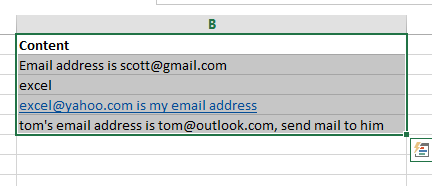#2 go to DATA tab, click Filter command under Sort & Filter group. One Filter arrow will be added in the first cell.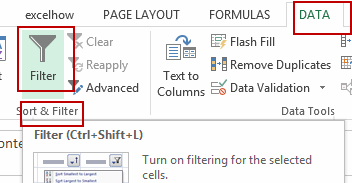#3 click the Filter arrow, and select Text Filters->Custom Filter from the sub menu list. And the Custom AutoFilter dialog will open.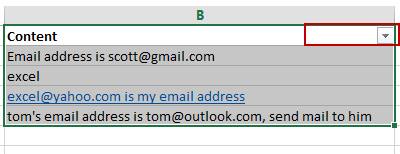#4 select criteria as Equals, and type 5 question marks as pattern mode. It indicates that it will match the text string that the length is 5. Click Ok button.#5 let’s see the result.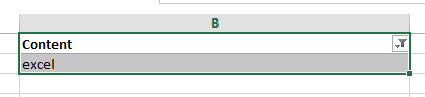## Filtering Cells by Text Length with a Formula

You can also create a help column in combination with a formula based on the LEN function to achieve the result of filtering cells by text length in Excel. Just do the following steps:

#1 select one adjacent column, such as column C, and type the following formula into cell C2. And press Enter key. And then drag the AutoFill Handle over other cells to apply this formula.

=LEN(B2)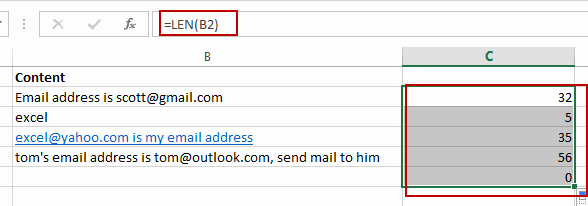#2 select the Column C, and go to DATA tab, click Filter command under Sort & Filter group. One Filter arrow will be added in the first cell.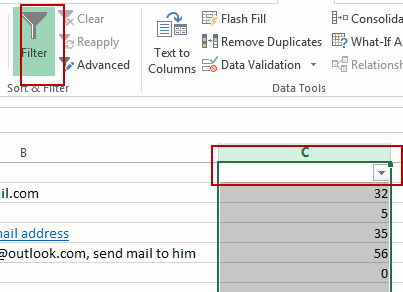#3 click the Filter arrow, and uncheck the Select All check box, and select one length number, click OK button.

#4 let’s see the result.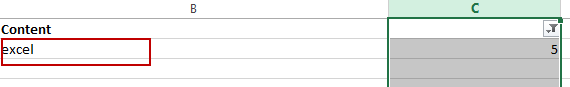Related Posts
If Cell is This Value or That Value

IF function is frequently used in Excel worksheet to return you expect “true value” or “false value” based on the result of logical test. If you want to see if a cell is A or B, and if one of ...

If Value is Greater Than A Certain Value

IF function is frequently used in Excel worksheet to return you expect “true value” or “false value” based on the logical test result. If you want to see if a value in one cell is greater than a specific value, ...

If Cell is Not Blank

IF function is frequently used in Excel worksheet to return you expect “true value” or “false value” based on the result of created logical test. If you want to see if a cell is blank or not, and leave some ...

VBA Macro For VLOOKUP From Another Sheet

In the previous post, you should know that how to fix or remove the #N/A error when using VLOOKUP formula to lookup value from another sheet. And this post will show you how to use VBA code to vlookup data ...

If Cell is Blank

IF function is frequently used in Excel worksheet to return you expect “true value” or “false value” based on the result of created logical test. If you want to see if a cell is blank or not, and leave some ...

If Cell Equals Certain Text String

IF function is frequently used in Excel worksheet to return you expect “true value” or “false value” based on the result of created logical test. If you want to see if cell equals a certain text string like “Win”, you ...

If Cell Contains Either Text1 or Text2

IF function is frequently used in Excel worksheet to return “true value” or “false value” based on the logical test result. If you want to see if cell contains certain substring1 like “abc” or substring2 like “def”, and returns true ...

If Cell Contains Certain Text OR Equals Certain Text

IF cell equals certain text IF function is frequently used in Excel worksheet to return “true value” or “false value” based on the logical test result. If you want to test values to see if they equal certain text like ...

VLOOKUP From Another Sheet Not Working

In the previous post, you should know that how to fix or remove the #N/A error when using VLOOKUP formula to lookup value from another sheet. And this post will show you reasons why your VLOOKUP formula is not working ...

If Cell Begins with One of Three Supplied Characters

If you want to test values to see if they begin with some given specific characters like “x”, ”y”, or “z”, you can create a formula with COUNTIF and SUM functions to return results. EXAMPLE You can see “TRUE” or ...

Sidebar